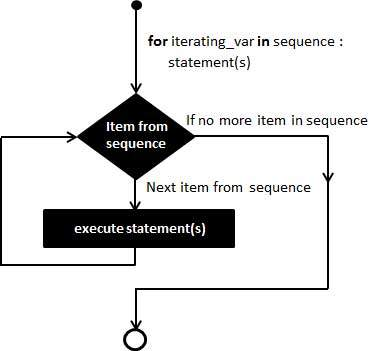# For Loop In Python

The for loop in Python has the ability to iterate over the items of any sequence, such as a list or a string.

## Syntax:

The syntax of a for loop look is as follows:
for iterating_var in sequence:
statements(s)If a sequence contains an expression list, it is evaluated first. Then, the first item in the sequence is assigned to the iterating variable iterating_var. Next, the statements block is executed. Each item in the list is assigned to iterating_var, and the statement(s) block is executed until the entire sequence is exhausted.

## Flow Diagram:## Example:

write a programs to print natural number from 1 to n terms.

## Example:

#!/usr/bin/python

for letter in 'Python': # First Example
print 'Current Letter :', letter

fruits = ['banana', 'apple', 'mango']
for fruit in fruits: # Second Example
print 'Current fruit :', fruit

print "Good bye!"When the above code is executed, it produces the following result:
Current Letter : P
Current Letter : y
Current Letter : t
Current Letter : h
Current Letter : o
Current Letter : n
Current fruit : banana
Current fruit : apple
Current fruit : mango
Good bye!

## Iterating by Sequence Index:

An alternative way of iterating through each item is by index offset into the sequence itself. Following is a simple example:
#!/usr/bin/python

fruits = ['banana', 'apple', 'mango']
for index in range(len(fruits)):
print 'Current fruit :', fruits[index]

print "Good bye!"When the above code is executed, it produces the following result:
Current fruit : banana
Current fruit : apple
Current fruit : mango
Good bye!
Here, we took the assistance of the len() built-in function, which provides the total number of elements in the tuple as well as the range() built-in function to give us the actual sequence to iterate over.

## The else Statement Used with Loops

Python supports to have an else statement associated with a loop statement.
If the else statement is used with a for loop, the else statement is executed when the loop has exhausted iterating the list.If the else statement is used with a while loop, the else statement is executed when the condition becomes false.The following example illustrates the combination of an else statement with a for statement that searches for prime numbers from 10 through 20.
#!/usr/bin/python

for num in range(10,20): #to iterate between 10 to 20
for i in range(2,num): #to iterate on the factors of the number
if num%i == 0: #to determine the first factor
j=num/i #to calculate the second factor
print '%d equals %d * %d' % (num,i,j)
break #to move to the next number, the #first FOR
else: # else part of the loop
print num, 'is a prime number'When the above code is executed, it produces the following result:
10 equals 2 * 5
11 is a prime number
12 equals 2 * 6
13 is a prime number
14 equals 2 * 7
15 equals 3 * 5
16 equals 2 * 8
17 is a prime number
18 equals 2 * 9
19 is a prime number